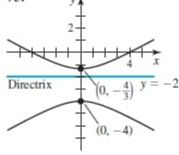# Get help with parametric equations

Recent questions in Parametric equationsbabeeb0oL 2021-09-09 Answered

### Find $$\displaystyle{\frac{{{d}^{{{3}}}{y}}}{{{\left.{d}{x}\right.}^{{{3}}}}}}$$ for the following parametric equation: $$x=t^{2}-3$$ $$y=t^{8}$$ $$t=2$$UkusakazaL 2021-09-09 Answered

### Convert the equation $$\displaystyle{\left({x}+{3}\right)}^{{{2}}}+{\left({y}—{5}\right)}^{{{2}}}={25}$$ into polar equation. Sketch the graph and identify the type of conic/shape.Efan Halliday 2021-09-09 Answered

### Transform polar function $$\displaystyle{r}=-{4}{\sin{{\left(\theta\right)}}}$$ to rectangular coordinates and graph, label your your graph clear with axes and scalesArmorikam 2021-09-08 Answered

### Convert each polar equation to rectangular form. $$\displaystyle=−\frac{\pi}{{6}}$$tinfoQ 2021-09-08 Answered

### Find the parametric equation for a circle with radius 3 and center at (−2, 5).Ava-May Nelson 2021-09-08 Answered

### Find the parametrization of the function $$\displaystyle{f{{\left({x}\right)}}}={2}{x}^{{{3}}}−{7}$$Harlen Pritchard 2021-09-08 Answered

### Convert the point $$(-1,1)$$ from Cartesian coordinates to polar coordinatesalesterp 2021-09-07 Answered

### The plane $$\displaystyle{x}+{y}+{2}{z}={18}$$ intersects the paraboloid $$\displaystyle{z}={x}^{{2}}+{y}^{{2}}$$ in an ellipse. Find the points on this ellipse that are nearest to and farthest from the origin.ringearV 2021-09-07 Answered

### Replace the polar equations with equivalent Cartesian equations. Then describe or identify the graph. $$\displaystyle{r}^{{{2}}}=-{6}{r}{\sin{\theta}}$$Cheyanne Leigh 2021-09-07 Answered

### Rectangular equation y=2 convert to polar equationTabansi 2021-09-07 Answered

### Consider the parametric equation $$\displaystyle{x}={2}{t}-{1},{y}={8}{t}^{{3}}.-{1}\le{t}\le{1}$$ a)Eliminate the parameter to obtain an equation in x and y. b)Sketch the graph of the equationUkusakazaL 2021-09-07 Answered

### Convert the point $$\displaystyle{\left({2},\frac{\pi}{{3}}\right)}$$ from polar coordinates to Cartesian coordinatesJaden Easton 2021-09-06 Answered

### Which of the following polar coordinate pairs does not represent the point with rectangular coordinates (-2,-2)? (A)$$\displaystyle{\left({2}\sqrt{{2}}.−{135}°\right)}$$ (B)$$\displaystyle{\left({2}\sqrt{{2}}.{225}°\right)}$$ (C)$$\displaystyle{\left(−{2}\sqrt{{2}}.−{315}°\right)}$$ (D)$$\displaystyle{\left(−{2}\sqrt{{2}}.{45}°\right)}$$ (E)$$\displaystyle{\left(−{2}\sqrt{{2}}.{135}°\right)}$$Efan Halliday 2021-09-06 Answered

### Change from rectangular to spherical coordinates. (Let $$\displaystyle\rho\geq{0},{0}\leq\theta\leq{2}\pi,\ \text{ and }\ {0}\leq\phi\leq\pi$$) (a) $$(0, -3, 0)$$ (b) $$\displaystyle{\left(-{1},{1},-\sqrt{{{2}}}\right)}$$Tammy Todd 2021-09-06 Answered

### Find a polar equation for the conic section. Assume one focus is at the origin.Kaycee Roche 2021-09-06 Answered

### Represent the line segment from P to Q by a vector-valued function $$P(-8,-2,-2), Q(-3,-9,-4)$$ Represent the line segment from P to Q by a set of parametric equationsEunoR 2021-09-06 Answered

### a) Express the polar equation in parametric from b) Graph the parametric equation you found in part a. $$\displaystyle{r}={2}^{{{\frac{{\theta}}{{{12}}}}}},{0}\leq\theta\leq{4}\pi$$Lipossig 2021-09-05 Answered

### The letters $$\displaystyle{r}{\quad\text{and}\quad}θ$$ represent polar coordinates. Write each equation using rectangular coordinates $$(x, y)$$. $$\displaystyle{r}= \sin{\theta}- \cos{\theta}{r}= \sin{\theta}- \cos{\theta}$$ Help, please.Carol Gates 2021-09-05 Answered

### Convert the Cartesian coordinates $$(-5,-12)$$ into polar coordinates where $$r >0$$ and $$\displaystyle{0}^{{{o}}}\leq\theta\leq{360}^{{{o}}}$$. Hence, write the other two polar coordinates that represent the point.Haven 2021-09-05 Answered

...